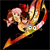## Want to see ALL questions on this topic?

### Upgrade to PLUS+ for €35 to see all past questions## You need to have an account to continue

3 Commentswhen adding or subtracting fractions, the denominator must be the same eg. 2/3 + 2/6.... find lowest common denominator (LCD) which is 6... 2/3... 6 goes into 3 two times so 2*2/2*3 = 4/6.........2/6...6 goes into 6 once so 1*2/6+ 2/6 now that the denominators are equal u can add but add only the numerator so 4/6 +2/6 = 6/6 which equals 1for multiplying its normal multiplicaton ... 2/3 * 2/6.... numerator* numerator and denominator*denominator.... 2*2/3*6 = 4/18.. simplify if necessary =2/9for division u leave the first fraction and flip the second and turn division to multiplication eg. 2/3 divided by 2/6.... leave 2/3 as it is but flip the 2/6 and turn to multiplication so now ur equation is 2/3 * 6/2 = 12/6 =2Uploading attachment...